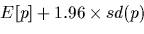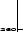Next: Molecular Weight Traces Up: Searching with Fragment Sequences Previous: Generating random scores

# Computing expected scores

We can compute the exact expected value of a random name matching the given fragment if we ignore deletions. Ignoring deletions is not totally out of the question, as deletions will not occur very often in short fragments. For example, when we match amino acid G against a random amino acid, the expected score is given by:and its varianceFor a sequence of amino acids a1, a2, ..., anthe expected score and variance areand. The boundary for the score can be computed now from the expected value and variance as we did in the previous section. The function AvgRandomSimil does all these computations together. The only difference is that now we are considering a match for every possible amino acid in the database, and hence to expect 10 random matches the goal should be set to go give a probability of 10/m where m is the number of amino acids in the database.
> AvgRandomSimil := proc(Seq:string, DM:DayMatrix, prandom:real)
> D := DM[Sim]:
> Dsq := copy(D):
> for i to 20 do for j to 20 do Dsq[i,j] := D[i,j]^2 od od;
> E := D*AF;
> V := Dsq*AF - zip(E*E);

Notice that E and V can be viewed as vectors, and are the result of multiplying the matrix  D times the frequency vector AF. The zip  operation works on vectors  and returns the vector of the product (square in this case) of each component.
> escore := vscore := 0;
> for i to length(Seq) do if Seq[i] <> 'X' then
>     j := AToInt(Seq[i]);
>     escore := escore + E[j];
>     vscore := vscore + V[j];
>     fi od;
> printf('Similarity against %s:\n',Seq);
> printf('Mean %.2f, variance %.2f \n', escore, vscore );
> printf('Probability of random match less than %g\n', prandom);
> goal := escore+sqrt(2*vscore)*erfcinv(2*prandom);
> printf('Goal should be %.1f\n', goal);
> end:

According to this derivation a minimum acceptable goal given by
> AvgRandomSimil( SearchSeq, DM, 10/DB[TotAA] );
Error, cannot apply selector on object

should be used. These theoretical expected value and variance are in full agreement with the experimentally measured value recorded above.
> print(ByDynProgr);
Error, (in Stats_print) division by zero


The two bounds,

Error, cannot convert to a number

are not very close for this example. This is because the two methods for estimating them are quite different. The random generation of alignments selects the best match out of a longer sequence. If our sequence has 9 amino acids and we are doing a LocalAlign against a random sequence with 300 amino acids, this would be equivalent to selecting the highest value of 292 possible exact matches. This alignment may include deletions which could improve the score. In any case, while both estimates are not very far apart, we should use the one based on the match sampling as it closely reflects our real computations.

Given these bounds on the similarity we are now able to run the search. This is done with the function AlignOneAll  with the following arguments:

> found := AlignOneAll(SearchSeq,DB,DM,Goal):
Error, wrong number (or type) of parameters in function AlignOneAll;

> length(found);
5

The result of AlignOneAll is a set with 5 matches. So far all the computations were made with the Dayhoff matrix DM, which is the standard 250-PAM matrix. At this point we want to refine these matches by computing an estimate of their PAM distance.  This is done with the function LocalAlignBestPam . Additionally every match with a similarity score lower than 60 will be ignored. The following loop does the LocalAlignBestPam refining and compresses the result to the left of the list keeping just those with high enough similarity.
> j := 0:
> for i to length(found) do
>     found[i] := LocalAlignBestPam(found[i]);
>     if found[i,Sim] >= 60 then
>         j := j+1;
>         found[j] := found[i]
>         fi
>     od:
LocalAlignBestPam expects a 1st argument, m:Match, found: f
Error, invalid arguments

> found := found[1..j]:
> length(found);
0

We are now left with 0 matches which are likely to be significant. To facilitate their inspection we can sort them in decreasing order of similarity:
> found := sort(found,x -> -x[Sim]):
Error, invalid arguments to sort

The two most significant matches are identities:
> print( op(found[1..2]) );
Error, range selector out of bounds

and the two least significant are:
> print( op(found[-2..-1]) );
Error, range selector out of bounds

Please notice that if we were interested in identities alone, the function SearchSeqTagDb  described in chapter 8 should have been used.

All the functionality described above is encapsulated in a single function called SearchFrag . SearchFrag takes a fragment name as an argument and returns the set of refined matches with a similarity score 70 or higher. This similarity bound can be altered by setting MinSim  to the desired value. SearchFrag computes the minimum score such that about 10 random matches will be reported, which appears to be satisfactory for most situations. For example, all the above computations can be done with

> MinSim := 60:
> found := SearchFrag( GKSKGFGFV ):
Error, (in SearchFrag)
A peptide database must be loaded before running SearchFrag

Minor differences in values are due to taking random samples which will not be the same for the two runs.

Enough information now exists to perform an all against all matching of the polypeptides that contain the sequence of interest, thus allowing the discovery of homologies among the complete sequences picked up by the fragment. The functional subprogram SmallAllAll  does the actual cross referencing. SmallAllAll requires that the sequences of interest be copied into a file or database of their own.

The following code extracts the peptides containing a match into a file called SubSwiss'.

> WriteFile('SubSwiss'):
> printf('<DBDESCR>Entries matching the peptide\n');
> printf('fragment %s </DBDESCR>\n',SearchSeq);
> for m in found do print(Entry(GetEntryNumber(m[Offset1]))) od;
> WriteFile('terminal'):

The sub-database is now ready to be loaded for the SmallAllAll.
> ReadDb('SubSwiss');
Reading 107 characters from file SubSwiss
Pre-processing input (DNA)
0 sequences within 0 entries considered
Error, database contains no entries

Notice that now the default peptide database contains just
Error, cannot apply selector on object
`
sequences as it should. The only remaining job is to run an all against all match of the sub-database. A complete description of the SmallAllAll procedure appears in Chapter.Next: Molecular Weight Traces Up: Searching with Fragment Sequences Previous: Generating random scores
Gaston Gonnet
1998-09-15